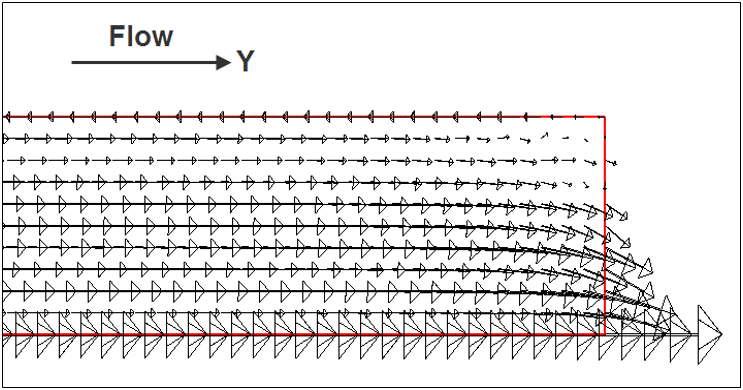## Model Response to Coriolis Forcing

This is an analytical test for the accuracy of coriolis force generated three-dimensional current and water surface slope.

Domain

• Cuboid flume
• 100,000 m length
• 5,000 m width
• 1 m height
• 45 degree latitude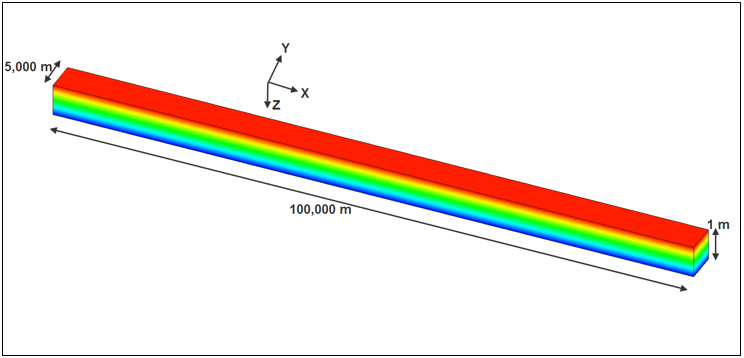Initial Conditions

• Water depth of 1 meter

Boundary Conditions

• No time varying boundary conditions

Results

• Run simulation to steady state
• Analytic solution for x direction flow
• Assume uniform flow
• y velocity = 0.0
• Change in u velocity with respect to x = 0.0
• Therefore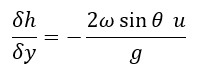= -2.0988e-6 m/m

• AdH computed slope = -2.154e-6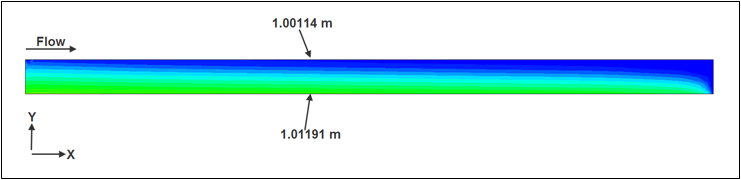• Analytic solution for y direction flow​
• Run simulation to steady state
• Assume uniform flow
• x velocity = 0.0
• Change in v velocity with respect to y = 0.0
• Therefore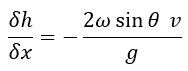= -2.0988e-6 m/m

• AdH computed slope = -2.000e-6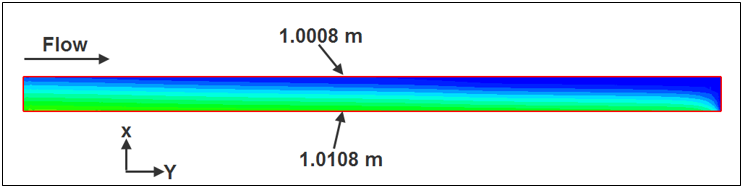• Velocity shows a curvature to the right which is again supported by Coriolis theory